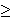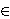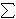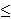# Approximating the Set Cover ProblemI just finished my weekly task of shopping for groceries. This can be a somewhat daunting task because I generally have a list of things that I’ll need which cannot all be purchased at a single location. What often happens is that I find that many of the items on my list are ONLY offered at certain stores – generic brands of certain items for example. My goal then changes from minimizing the total amount of money spent to minimizing the number of stores that I must visit to purchase all of my items.

To formulate this as a mathematical problem, suppose that I have a grocery list of items I would like to buy, represented by the lists item1, item2, …, itemn, where n represents the number of items I have on this list. Suppose also that there are stores Store1, Store2, …, Storem (each one distinct) that offer some combination of items I have on my list. What I would like to do is minimize the number of stores I have to visit to purchase these items.

The problem I just described is famous because it is one that many people face on a regular basis. In a more general form, it is so famous that it has a name for it, called the Set Cover Problem (or the Minimum Set Cover Problem). In the general form of this problem, we replace the grocery list with a set of items called our universe. The lists of items offered at each store are the collections of subsets of the universe. In the problem, as in the example above, we would like to select enough subsets from this collection that we are able to obtain every element in our universe. We would like to do this with as low a number of sets as possible.

In my previous post, I described the 21 problems that Karp proved were NP-Complete. Set Cover was one of those problems, showing that this is a hard problem to solve. What I will do is introduce three ways to reach a near-optimal solution relatively quickly.

Greedy Method

One of the first approaches one may take to solve this problem is to repeatedly select the subset that contains the most new items. That’s how the greedy approach to set cover operates. The method knows to terminate when all elements belong to one of the selected sets. In the shopping example above, this would be accomplished by visiting the store that had the most items on my list and purchasing those items at this store. Once this is done, the items that have been purchased can be crossed off my list and we can visit the store with the most items on my remaining list, stopping when the list is empty.

Linear Programming Relaxation

Instead of stating the set cover problem with words, there is a way of describing the situation with mathematical inequalities. For instance, suppose that the soap I like to purchase is only available at stores Store1, Store4 and Store9. Then I could introduce a variable xi for each store i and the requirement that I purchase this soap can be restated as :

x1 + x4 + x91

Because we can either purchase some items or not purchase these items, each variable xi is 0 or 1 (called a binary variable). We can introduce similar constraints for each element in our universe (or on our grocery list). These inequalities (called constraints) have the form:

for each element eU,i | eSi xi1

Our goal of minimizing the number of sets chosen (stores visited) can be stated by the objective function:
minimize1in xi

So the mathematical formulation for this problem can be stated as

minimize1in xi
Subject to
for each element eU,i | eSi xi1
for each set i, xi{0, 1}.

Formulations of this type, where variables are restricted to a finite set (in this case the x variables being either 0 or 1) are called integer programs. Unfortunately, there is no easy way to solve these formulations either. However, there is a related problem which can be solved quickly.

Instead of restricting the x variables to the values of 0 or 1, we could allow them to take on any value within this range, i.e. 0xi1 for each set Si. Doing this converts the problem from an integer programming problem into a linear programming problem (called the LP-Relaxation), which can be solved quickly. The issue with this method though is that the solution obtained by an LP-Relaxation is not guaranteed to be an integer. In this case, how do we interpret the values xi?

Randomized Rounding Method

One approach to dealing with a non-integer solution to the LP-Relaxation is to treat the xi values as probabilities. We can say that xi is the probability that we select set i. This works because each value of xi is in the range of 0 to 1, which is necessary for a probability. We need to repeatedly select sets with their associated probabilities until all elements in our universe are covered. Selecting our sets based on this procedure is the randomized rounding approach.

Deterministic Rounding Method

A second approach to dealing with a non-integer solution to the LP-Relaxation is to base our solution on the most occurring element. If we let f be this frequency (i.e.the number of sets that the most occurring element occurs in), then we can define a solution by selecting set i if the LP=Relaxation solution gives the variable xi a value of at least (1/f).

None of these three approaches is guaranteed to give an optimal solution to an instance of this problem. I will not go into it in this post, but these can all be shown to be within some guaranteed range of the optimal solution, thus making them approximation algorithms.

Hope you enjoy.

# Knapsack ProblemsTo help understand this problem, I want you to think about a common situation in many people’s lives. You have a road trip coming up today and you’ve overslept and are at risk of missing your flight. And to top matters off, you were planning to pack this morning but now do not have the time. You quickly get up and begin to get ready. You grab the first bag you see and quickly try to make decisions on which items to take. In your head you’re trying to perform calculations on things you’ll need for the trip versus things that you can purchase when you get there; things that you need to be able to have a good time versus things you can do without. And to top matters off, you don’t have time to look for your ideal luggage to pack these things. So you have the additional constraint that the items you pick must all fit into this first bag you found this morning.

The situation I described above is a common problem. Even if we ignore the part about the flight, and just concentrate on the problem of trying to put the most valuable set of items in our bag, where each item has its own value and its own size limitations, this is a problem that comes up quite often. The problem is known (in the math, computer science and operations research communities) as the knapsack problem. It is known to be difficult to solve (it is said to be NP-Hard and belongs to a set of problems that are thought to be the most difficult problems within its class). Because of this difficulty, this problem has been well studied.

What I provide in my script are two approaches to solving this problem. The first is a greedy approach, which selects a set of items by iteratively choosing the item with the highest remaining value to size ratio. This approach solves very fast, but can be shown to give sub-optimal solutions.

The second approach is a dynamic programming approach. This algorithm will solves the problem by ordering the items 0, 1, …, n and understanding that in order to have the optimal solution on the first i items, the optimal solution must have been first selected on the fist i-1 items. This algorithm will optimally solve the problem, but it requires the computation of many sub-problems which causes it to run slowly.

Update (4/2/2013): I enjoy this problem so much that I decided to implement two additional approaches to the problem: Linear Programming and Backtracking.

The Linear Programming approach to this problem comes from the understanding that the knapsack problem (as well as any other NP-Complete problem) can be formulated as an Integer Program (this is a mathematical formulation where we seek to maximize a linear objective function subject to a set of linear inequality constraints with the condition that the variables take on integer values). In the instance of the knapsack problem we would introduce a variable xi for each item i; the objective function would be to maximize the total value of items selected. This can be expressed as a linear objective function by taking the sum of the products of the values of each item vi and the variable xi; the only constraint would be the constraint saying that all items fit into the knapsack. This can be expressed as a linear inequality constraint by taking the sum of the products of the weights of each item wi and the variable xi. This sum can be at most the total size of the knapsack. In the integer programming formulation, we either select an item or we do not. This is represented in our formulation by allowing the variable xi = 1 if the item is selected, 0 otherwise.

The LP relaxation of an integer program can be found by dropping the requirements that the variables be integer and replacing them with linear equations. So in the case of the knapsack problem, instead of allowing the variables to only take on values of 0 and 1, we would allow the variables to take on any value in the range of 0 and 1, i.e 0 <= xi <= 1 for each item i. We can then solve this LP to optimality to get a feasible solution to the knapsack problem.The second knapsack approach I implemented today is through backtracking. Similar to the Dynamic Programming approach to this problem, the backtracking approach will find an optimal solution to the problem, but these solutions generally take a long time to compute and are considered computationally inefficient. The algorithm I implemented here first orders the item by their index, then considers the following sub-problems for each item i "What is the best solution I can obtain with this initial solution?". To answer this question, the algorithm begins with an initial solution (initially, the empty set) and a set of unchecked items (initially, all items) and recursively calls itself on sub-problems with an additional item as a part of the initial solution and with this item removed from the unchecked items.So check out my knapsack problem page. I think its a good way to be introduced to the problem itself, as well as some of the techniques that are used in the fields of mathematics, computer science, engineering and operations research.

Other Blogs covering this topic:
Journey to the Four Point Oh

# The Simplex Method

I just added a script which generates a random linear programming problem and executes the Simplex Method Method on it.

The Simplex Method, originally discovered by George Dantzig, is one of the most important algorithms in computer science. It literally connects computer scientists to problems in mathematics, engineering, business, economics, transportation, and a host of problems that are faced by everyday people. For many of these problems, no one has yet discovered a pattern or a simpler means of solving it, other than the Simplex Method. Each of these problems has its own difficulties that distinguish it from other problems. While some such problems are important enough to receive their own studies to improve upon the efficiency of the simplex method (Network Flow problems come to mind), there are just too many problems for each one to receive such consideration. Thus we see the importance of the Simplex Method because it can solve these problems as long as they can be formulated as linear programming problems.

To understand the power of the Simplex Method, we need to understand a class of problems: linear programming problems. A linear programming problem is a problem where we seek to minimize/maximize a linear objective function (over finitely many variables) subject to a finite set of linear inequality constraints over these variables. A general example of a linear programming problem is “The Transportation Problem”.

The Transportation Problem Suppose you own a manufacturing company and its your job to ship your product from warehouse locations all across the United States to a number of customers. Each customer has ordered a certain quantity of the item, and each warehouse can supply up to a certain amount. Then we define the following variables:

m = the number of warehouses
n = the number of customers
ai = the total amount of item available at warehouse i
bj the total requirement of customer j
xi, j = the amount of item shipped from warehouse i to customer j.

In order for things to work properly, we need that the total amount in supply is equal to the the total amount ordered. If we assumed that we have 3 customers and 2 warehouses, then the following constraints help formulate this problem.

x1, 1 + x1, 2 + x1, 3 = a1
x2, 1 + x2, 2 + x2, 3 = a2
x1, 1 + x2, 1 = b1
x1, 2 + x2, 2 = b2
x1, 3 + x2, 3 = b3

You also know the cost of shipping one unit of the item from warehouse i to customer j and label it ci, j.

Then the objective function which we seek to minimze is c1, 1x1, 1 + c1, 2x1, 2 + c1, 3x1, 3 + c2, 1x2, 1 + c2, 2x2, 2 + c2, 3x2, 3.

And we also have that xi, j is nonnegative since we are shipping (and not receiving) goods.

Because we can state this problem as a linear programming problem, we can solve it using the simplex method. Although this problem was very simple to formulate, the ability to formulate a problem as a linear programming problem is seen as a great accomplishment because there exists so much literature on linear programming problems, and a method on how to solve them, (i.e. the Simplex method). For this reason, much work is often dedicated to finding good linear programming representations of problems.

Another set of problems of interest are integer programming problems. These problems add the additional requirement to linear programming problems that some or all of these variables can only take on a discrete set of values instead of a continuous realm. Integer programming problems are generally thought to be more difficult to solve than linear programming problems. What makes integer programming problems special is the fact that many problems that are known to be just as difficult as integer programming problems can be formulated as integer programming problems. Although the Simplex Method does not promise to always solve these problems to optimality, it offers a way to approach integer programming problems, and thus every problems that is known to be just as hard these problems which is at least a starting point.

# New Years is a LEARNINGlover Thing!

Starting this web site has served as the perfect opportunity to unwind. In particular, two blogs I wrote recently have served different purposes. The first was “The Degrees of Consciousness of a Black Nerd“, where I spoke about many of the things I think about being who I am and relating the the (somewhat unique) set of people that I communicate with on a daily basis. The other was what I’ve been working on since mid December. Its the blog entry I wrote on Sudoku and the Sudoku program that I wrote last month using the Dancing Links Algorithm. Since originally writing that, I’ve updated the program with a lot of Sudoku problems, as well as two types of “hints”. One generates the “possibilities matrix” which basically just shows what is possible for each cell. The other scans the possibilities matrix and searches for isolated cells (cells where some number can only go in one row/column/subgrid). Both those additions were extremely fun and provided a nice opportunity to program in my spare time.

So I’ve been thinking about these two things and how much I enjoyed the two, but for different reasons. The Degrees of Consciousness of a Black Nerd brought much attention on facebook where the idea was both accepted and rejected and I was able to explain the ideas further and hear similar stories from others who had similar experiences. Sudoku, on the other hand hasn’t generated as much conversation. A few friends have told me that they liked the program, but I don’t know of too many people outside of myself using it. That’s OK. I didn’t really create the program for publication, moreso because I enjoy Sudoku and took it as a challenge to write a program to solve a puzzle.

That being said, I’ve been thinking about some other things that I would like to do – hopefully they’ll be more than just my opinion, but have some programming, operations research, or mathematical context to them as well. But here’s a list of things that I want to write about in the near future.

• Connections between math and football (or sports in general). Anybody who knows me knows that I’m a huge sports fan. At other sites, I’ve posted stuff on QB rating systems and the flaws/inaccuracies in them. Part of me would like to look further into this stuff and either do a comparison, create a new rating system, or just try to understand (explain) different scouting metrics, particularly for QBs.
• I wrote the Sudoku program, but that’s a well studied problem so it was easy to find research that helped me to understand other stuff. I also studied some problems in Ramsey Theory that can be represented by exact covering algorithms and it would be interesting to try to represent these as exact cover problems and to try to use the Dancing Links algorithm to solve these problems as well, maybe to find a comparative analysis to benchmarks.
• One of the things I’ve picked up on lately is machine learning. While I’ve added flash cards on Bayesian Networks, I would like to add some programs on things like k-means clustering.
• I added my sorting algorithms a few weeks ago, but would like to also add something on data structures (arrays, linked lists, trees, heaps, hash tables, etc). In undergrad this was called the class that weeded people out of computer science majors, so doing something on this type of stuff I think could be helpful to those who want to understand it better.
• I have been in the process of writing a linear programming (Simplex) implementation for a while. I would like to get back to that to allow for a solver that can do at least simple algorithms.
• I’ve written a few drafts that connect math to different areas that I’m interested in (music, sports, philosophy, religion, etc), but I need to find a better way to present the stuff because as they’re currently stated, this stuff can easily be misinterpreted.

The beautiful thing about this site is that I’m not constrained by any advisor or boss or deadlines. Its more of a how am I feeling right now kind of a thing and these are the things I’m feeling right now. So this list is kind of my “New Years Resolutions” for LEARNINGlover.com, and while I reserve the right to change my priorities any time I feel that something else deserves my attention more, these are the things I’m planning to spend time on in the next few weeks.